﻿ 基于中压载波的10kV线路特性研究方法 Study on 10 kV Line Characteristics Based on Medium Voltage Carrier

Smart Grid
Vol. 10  No. 04 ( 2020 ), Article ID: 36852 , 7 pages
10.12677/SG.2020.104017

Study on 10 kV Line Characteristics Based on Medium Voltage Carrier

Yongbin Li1, Wenjun Chen1, Tao Yu1, Minglu Qi1, Wang Zhang1, Liang Li2

1State Grid Qinghai Electric Power Company, Guoluo, Xining Qinghai

2Qingdao Topscomm Communication Co. Ltd., Qingdao Shandong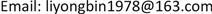Received: Jul. 1st, 2020; accepted: Jul. 27th, 2020; published: Aug. 3rd, 2020ABSTRACT

Using medium voltage carrier communication to achieve full coverage of power information acquisition and distribution automation has become a hot spot in the domestic power industry. The characteristics of 10 kV line will largely affect the reliability of medium voltage carrier communication, but due to the influence of voltage level and other factors, the research on 10 kV line characteristics is not mature. In this paper, a research method of 10 kV line characteristics based on medium voltage carrier is proposed. The improved medium voltage carrier communication machine and coupler can realize the collection of 10 kV line impedance, attenuation and noise, accumulate useful data for the long-term study of 10 kV line characteristics, help to improve the reliability of medium voltage carrier wave communication and assist 10 kV line fault monitoring.

Keywords:Medium Voltage Carrier, Carrier Machine, Coupler, Impedance, Attenuation, Noise1国网青海省电力公司果洛供电公司，青海 西宁

2青岛鼎信通讯股份有限公司，山东 青岛1. 引言

10 kV线路特性  是影响中压载波通信的主要因素，因此研究10 kV线路特性–阻抗、衰减、噪声格外重要，但受到电压等级等因素的影响，目前对10 kV线路特性的研究更多局限于仿真建模、高精尖试验设备现场测试，实时性差且无法批量工程化应用，文中针对上述10 kV线路特性研究困难的问题，提出了一种基于中压载波  的10 kV线路特性研究方法，通过改进的中压载波通信设备实现对10 kV线路阻抗、衰减、噪声的采集，通过实际应用测试验证，证实了该方法能实现对10 kV线路特定频点下的阻抗、特定频点下线路区间衰减、特定频带范围内噪声的采集，可为10 kV线路特性长期研究积累有用数据，有助于提高中压载波通信可靠性，辅助10 kV线路故障监测，对于推动用电信息采集全覆盖和配电自动化建设具有重大意义。

2. 设备简介

2.1. 改进的中压载波通信机

2.2. 中压载波耦合器

3. 工作原理

3.1. 阻抗采集

10 kV线路阻抗  采集是基于改进的中压载波通信机实现特定频点下载波信号发送端和载波信号接收端10 kV线路的阻抗计算，原理框架图3所示。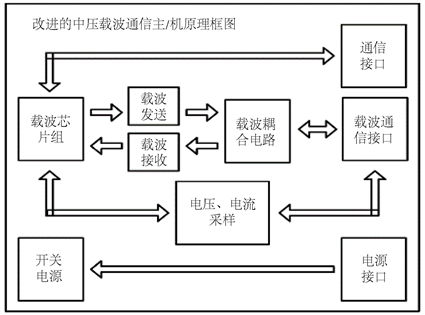Figure 1. Principle block diagram of improved medium voltage carrier communication machine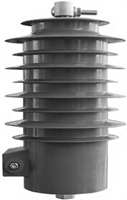Figure 2. Figure of coupler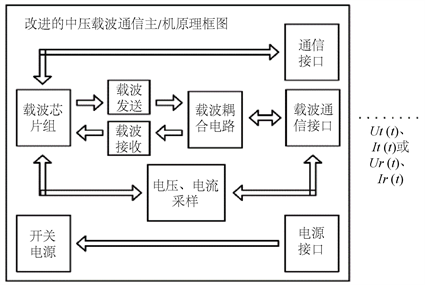Figure 3. Block diagram of 10 kV line impedance acquisition principle

${Z}_{t}\left(t\right)={U}_{t}\left(t\right)/{I}_{t}\left(t\right)$ (1)

${Z}_{r}\left(t\right)={U}_{r}\left(t\right)/{I}_{r}\left(t\right)$ (2)

3.2. 衰减采集

10 kV线路衰减  采集是基于改进的中压载波通信机实现特定频点下载波信号发送端与载波信号接收端之间10 kV线路的衰减计算，原理框图如图4所示：Figure 4. 10 kV line attenuation acquisition principle block diagram

$A=20|\mathrm{log}\left({U}_{s}\left(t\right)/{U}_{m}\left(t\right)\right)|$ (3)

3.3. 噪声采集

10 kV线路噪声采集是基于改进的中压载波通信机实现特定频带范围内载波信号发送端和载波信号接收端10 kV线路噪声采集，原理框图如图5所示：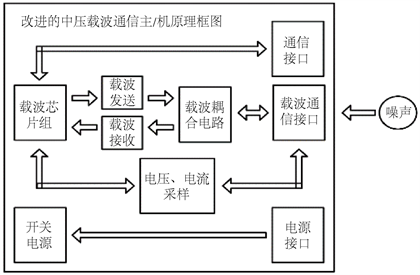Figure 5. 10 kV line noise acquisition principle block diagram

${b}_{k}=\frac{2}{N}\left[{\sum }_{i=0}^{N-1}f\left({T}_{0}+i\frac{T}{N}\right)\mathrm{sin}\left(2\pi \frac{i}{N}\right)-f\left({T}_{0}+\left(i-N\right)\frac{T}{N}\right)\mathrm{sin}\left(2\pi k\frac{i}{N}\right)\right]$ (4)

${a}_{k}=\frac{2}{N}\left[{\sum }_{i=0}^{N-1}f\left({T}_{0}+i\frac{T}{N}\right)\mathrm{cos}\left(2\pi \frac{i}{N}\right)-f\left({T}_{0}+\left(i-N\right)\frac{T}{N}\right)\mathrm{cos}\left(2\pi k\frac{i}{N}\right)\right]$ (5)

4. 现场测试

4.1. 阻抗测试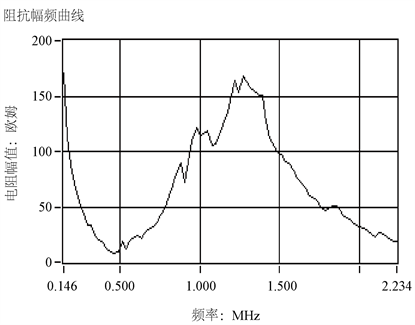Figure 6. Impedance distribution diagram collected by professional impedance acquisition equipment

4.2. 衰减测试

4.3. 噪声测试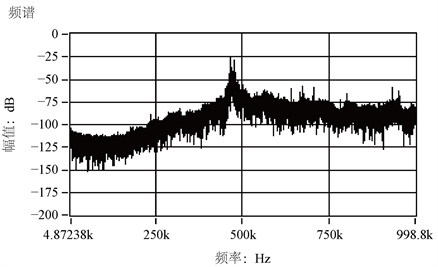Figure 7. Noise spectrum diagram collected by professional noise acquisition equipment

5. 结束语

Study on 10 kV Line Characteristics Based on Medium Voltage Carrier[J]. 智能电网, 2020, 10(04): 157-163. https://doi.org/10.12677/SG.2020.104017

1. 1. 陈辉, 马亚彬. 10kV电力线路的载波通信技术[J]. 自动化应用, 2017(4): 98-99, 104.

2. 2. 苟国栋. 中压载波在用电信息采集中的运用[J]. 科技创新与应用, 2017(35): 135-137.

3. 3. 车飞平. 配网自动化通信方案设计探讨[J]. 大众用电, 2016, 31(6): 28-29.

4. 4. 刘海涛, 宋健, 赵丙镇, 李晓. 中压电力线通信信道特性测试及分析[J]. 电力系统通信, 2006(4): 17-21.

5. 5. 妙红英, 王艳芹, 王松, 岳虎, 巨汉基, 刘岩, 洪虹, 康强. 基于中压电力线载波的通信技术研究[J]. 军民两用技术与产品, 2017(22): 216-218.

6. 6. 熊淦辉, 谢润生, 林伟坤. 架空线中压电力线载波的信道阻抗特性研究[J]. 电工技术, 2019(6): 71-73.

7. 7. 李丰. 低压电力线载波通信信道阻抗和衰减特性研究[D]: [硕士学位论文]. 保定: 华北电力大学, 2012.

8. 8. 王立城, 胡科军, 霍超, 李建岐. 低压电力线载波信道测试装置设计[J]. 电测与仪表, 2015(10): 44-49, 77.

9. 9. 胡苏阳, 王莉, 杨善水. 电力线信道系统噪声的建模方法研究[J]. 电测与仪表, 2018, 55(17): 88-93.

10. 10. 朱启贵, 王烨, 牟宪民. 10kV配电网电力线载波监测系统[J]. 电测与仪表, 2014(2): 10-13.